# Onsager-Machlup function

(diff) ← Older revision | Latest revision (diff) | Newer revision → (diff)
Jump to: navigation, search

Machlup–Onsager function

A function having its origin in physics and arising in a particular description of the dynamics of macroscopic systems. In this description the starting point is the calculation of a probability density for observing a complete path of a system in phase space spanned by the macroscopic variables. This approach was pioneered by L. Onsager and S. Machlup in [a1], who used this to develop a theory of fluctuations in (non-) equilibrium thermodynamics. Their work was restricted to the linear Gaussian case, which was subsequently extended to non-linear equations. This probability density can be expressed, apart from a normalizing factor, by means of a functional integral over paths of the process. The corresponding integrand has the form of the Lagrangian and has been called the Onsager–Machlup function by physicists.

R.L. Stratonovich [a2] first calculated this Onsager–Machlup function from a probabilistic viewpoint. The idea is to fix a smooth path in the state space, form a tube of small radius around this path and calculate asymptotically the probability of the sample paths of a diffusion lying within this tube. The most general result in this direction may be found in [a3]. Consider a Riemannian manifoldand suppose that a non-singular diffusion process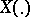is generated onby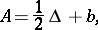whereis the Laplace–Beltrami operator (cf. Laplace–Beltrami equation) andis a vector field. Letwhere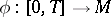,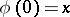, is a smooth curve onand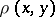is the Riemannian distance. For any two smooth curvesand,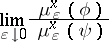exists and can be expressed as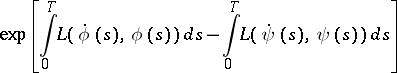for some functionalon the tangent bundle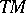. This function, which has the form of a Lagrangian (cf. also Lagrange function), is the Onsager–Machlup function. A detailed calculation forwhen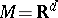may be founded in [a4].

The Onsager–Machlup function has recently (1990s) been applied to the non-linear filtering problem. Along with the development of the usual theory using conditional mean, various attempts have been made in the 1960s to heuristically calculate the conditional mode in the non-linear filtering problem (maximum a posteriori estimator). The mathematical difficulty arose due to the lack of a translation-invariant measure in function spaces. The first correct probabilistic interpretation of this filter has been given by A. Dembo and D. Zeitouni [a5] using the Onsager–Machlup function. Maximizing this function may be interpreted as giving the most probable trajectory, which is a natural generalization of the maximum-likelihood principle in statistics (cf. also Maximum-likelihood method). An extension of this approach to the estimation (smoothing) problem for random fields (cf. also Random field) may be found in [a6].